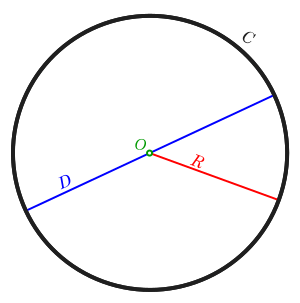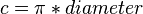# Circumference facts for kids

Kids Encyclopedia FactsCircumference (C in black) of a circle with diameter (D in cyan), radius (R in red), and centre (O in magenta). Circumference = π × diameter = 2 × π × radius.

In geometry, circumference is the distance around a closed curve; for example, a circle. It is a special kind of perimeter.(c=PI x diameter)$c = \pi * diameter$

## Images for kidsIn Spanish: Circunferencia para niños